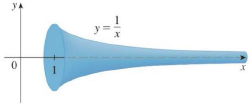Chapter 8.2, Problem 27E

Chapter
Section
Textbook Problem

If the region R = { ( x , y ) | x ≥ 1 ,     0 ≤ y ≤ 1 / x } is rotated about the x-axis, the volume of the resulting solid is finite (see Exercise 7.8.63). Show that the surface area is infinite. (The surface is shown in the figure and is known as Gabriel’s horn.)To determine

To show: The surface area obtained by rotating the region about x-axis is infinite.

Explanation

Given information:

The region is shown as follows:

={(x,y)|x1,0y1x}

The region is rotated about x-axis.

The curve is bounded between x=1 and x=.

The surface obtained by rotating the region about x-axis is called Gabriel’s Horn.

The volume of the resulting solid is finite.

Calculation:

Show the equation of the curve.

y=1x (1)

Calculate the area of the surface obtained by rotating the curve about x-axis using the relation:

S=ab2πy1+(dydx)2dx (2)

Here, S is the area of the surface obtained by rotating the curve about x-axis and

axb.

Differentiate both sides of Equation (1) with respect to x.

dydx=ddx(1x)=ddx(x1)=1x2=1x2

Substitute 1x2 for dydx, 1x for y, 1 for a, and for b in Equation (2).

S=12π1x1+(1x2)2dx=2π11x1+1x4dx=2π11xx4+1x4dx=2π1x4+1x3dx (3)

Consider the value of the function f(x)=x4+1x3

Consider the value of the function g(x)=1x.

Within the limits [1,]

x4+1x3>1xf(x)>g(x)

Show the definition of comparison theorem as shown below:

Suppose f and g are continuous function with f(x)g(x)0 for xa

Still sussing out bartleby?

Check out a sample textbook solution.

See a sample solution

The Solution to Your Study Problems

Bartleby provides explanations to thousands of textbook problems written by our experts, many with advanced degrees!

Get Started

In Exercises 31-34, evaluate h(2), where h = g f. 31. f(x) = x2 + x + 1; g(x) = x2

Applied Calculus for the Managerial, Life, and Social Sciences: A Brief Approach

For f(x)=7+5x2x2, find and simplify f(x+h)f(x)h

Mathematical Applications for the Management, Life, and Social Sciences

Find the mean for the following set of scores: 2, 7, 9, 4, 5, 3, 0, 6

Essentials of Statistics for The Behavioral Sciences (MindTap Course List)

2. Define the terms population, sample, parameter and statistic.

Statistics for The Behavioral Sciences (MindTap Course List)

The length of the are r = eθ for 0 ≤ θ ≤ π is given by:

Study Guide for Stewart's Multivariable Calculus, 8th